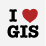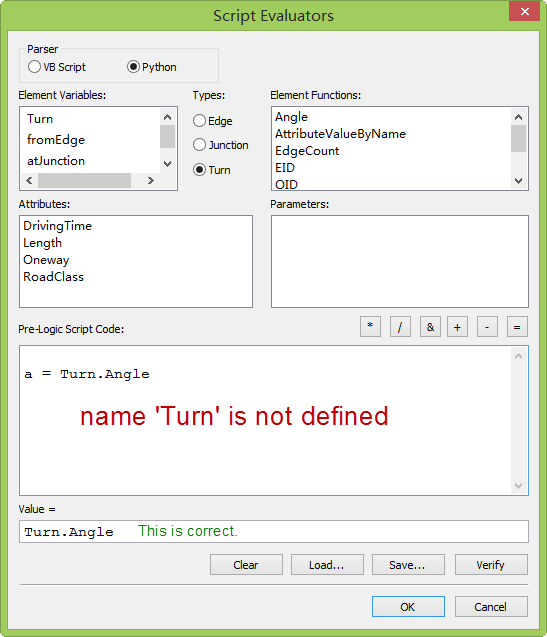# How to using python parser in "Script Evaluators" when element is Turn?

1064
3
11-29-2016 11:08 PMby
Occasional Contributor II

When calculate the turn value in the network dataset, is Turn.Angle only useful with VB Script? How to edit a pre-logic script code with turn.angle using python language?ArcMap Version: 10.4.0.5524

Tags (1)
1 Solution

Accepted SolutionsbyMVP Legendary Contributor

Code blocks in the normal sense, call a function using ! enclosing field names, I don't know if that is your case.  You didn't look far enough down in the examples in the link I sent to see their example... so check it out and see if this matches your case.

``````def somefunc(val):
if val.Angle > 270:
turnTime = 10
else:
turnTime = 20
return turnTime

# use this in the value= segment
somefunc(!Turn!)  # if Turn is a field‍‍‍‍‍‍‍‍‍‍``````
3 RepliesbyMVP Legendary Contributor

I don't understand your wrong section... you can't assign to a variable there.  The correct section, just takes the Turn and returns it's example.  More complex examples Types of evaluators used by a network—Help | ArcGIS for Desktop show a def being used and a field name enclosed in double exclamation marked being usedby
Occasional Contributor II

I want to add some logic for calculate the turn time with different turn angle.

For example:

This Script Evaluator expression is valid in VB Script:

If Turn.Angle > 270 Then
turnTime = 10
Else
turnTime = 20
End If

Value = turnTime

However this is not valid in python script.

if Turn.Angle > 270:
turnTime = 10
else:
turnTime = 20

Value = turnTimebyMVP Legendary Contributor

Code blocks in the normal sense, call a function using ! enclosing field names, I don't know if that is your case.  You didn't look far enough down in the examples in the link I sent to see their example... so check it out and see if this matches your case.

``````def somefunc(val):
if val.Angle > 270:
turnTime = 10
else:
turnTime = 20
return turnTime

# use this in the value= segment
somefunc(!Turn!)  # if Turn is a field‍‍‍‍‍‍‍‍‍‍``````